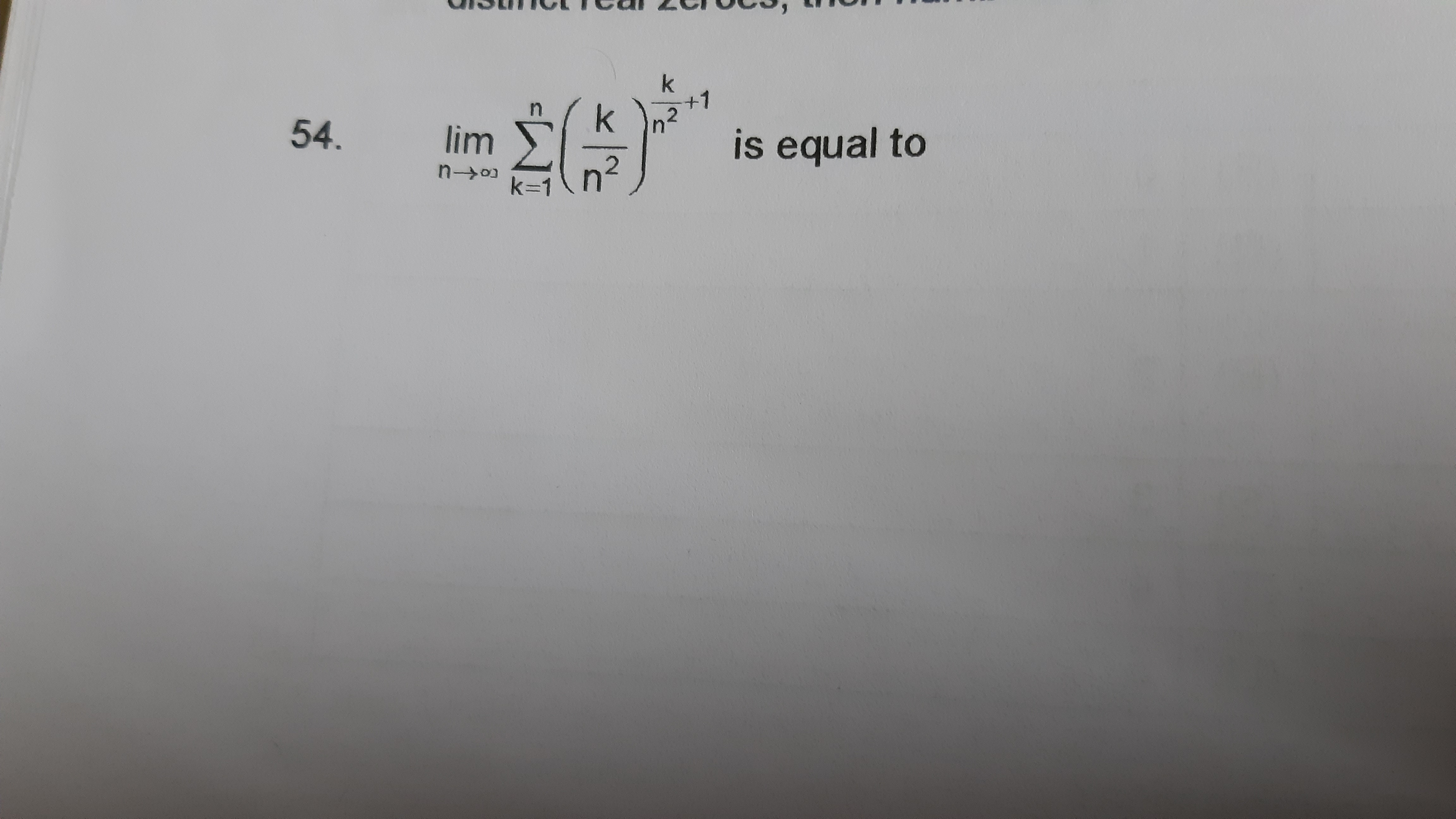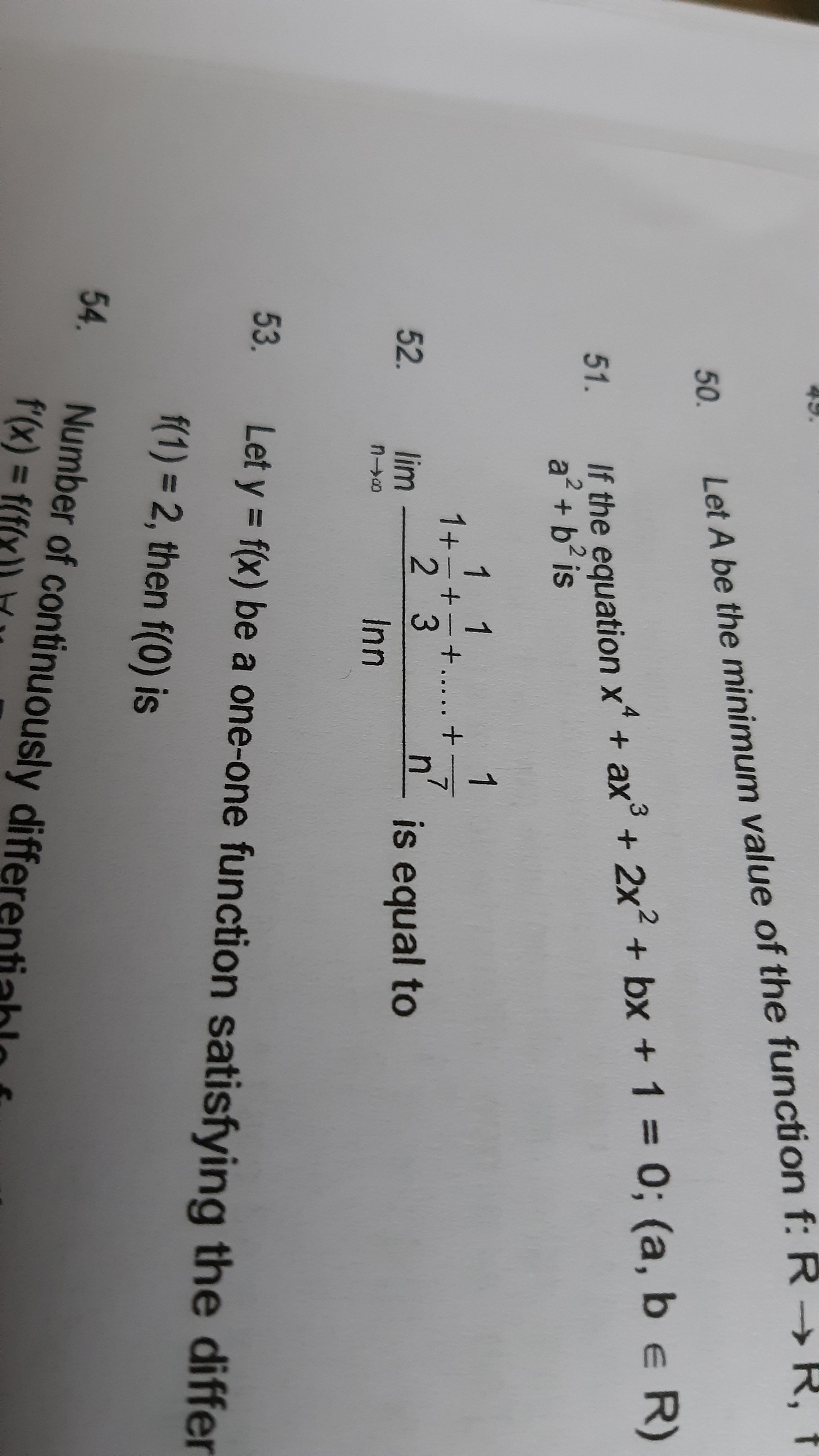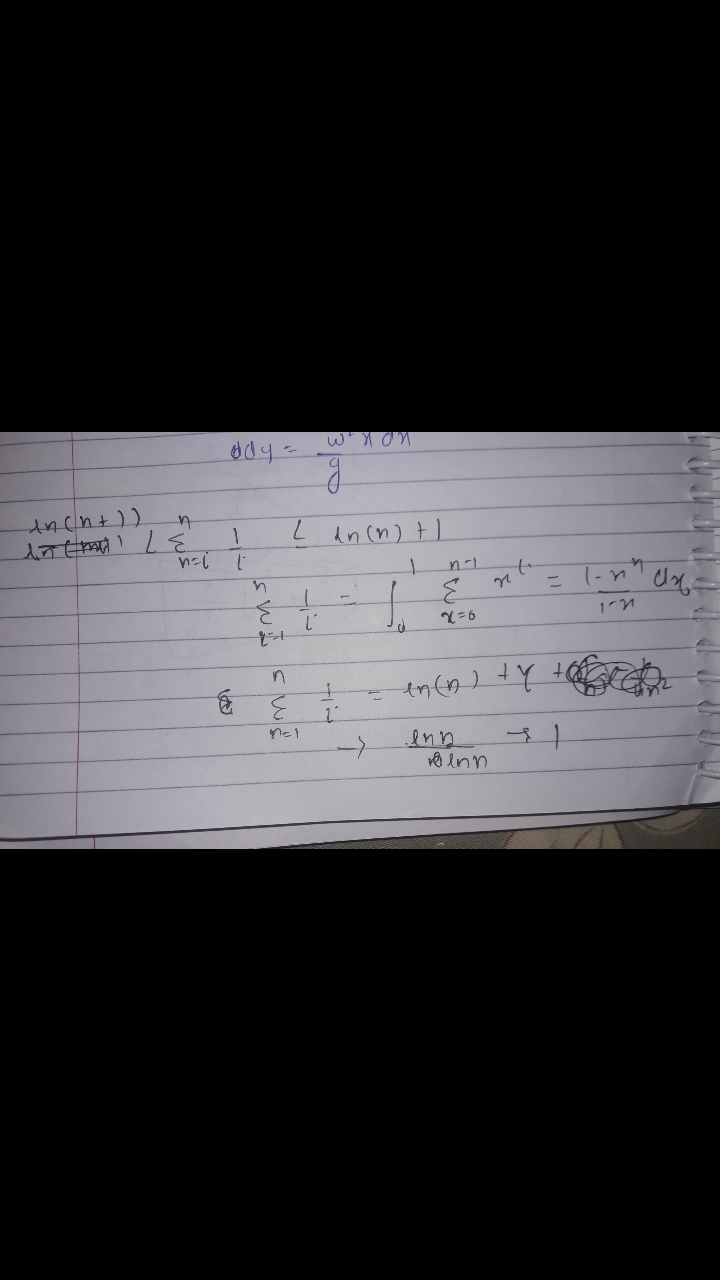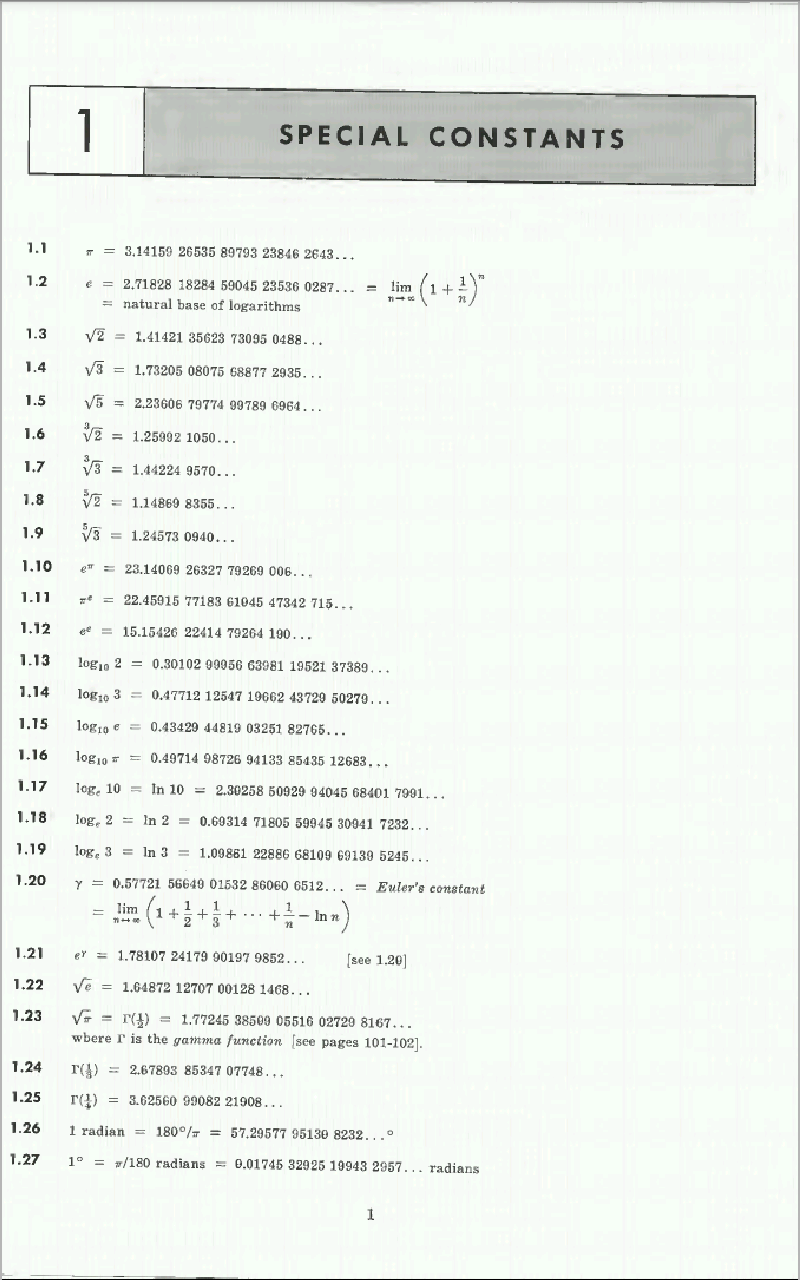# Doubt from limits

Question no 54, question 521 Like

54-i think may be zero for first i tried by stloz cesaro theoram @Hari_Shankar sir , @Jagdish_Singh sir what should be correct ans..

For (54)

\displaystyle \lim_{n\rightarrow \infty} \sum^{n}_{r=1}\bigg(\frac{r}{n^2}\bigg)^{\frac{r}{n^2}+1}=\frac{1}{2}.

For (52)

\displaystyle \lim_{n\rightarrow \infty}\frac{1}{\ln(n)}\bigg(1+\frac{1}{2}+\frac{1}{3}+\cdots +\frac{1}{n}\bigg)=1.

1 Like

for 52correct 1 is ans..

Yes sir we need solution too

#52: We have that 1+\dfrac{1}{2}+\cdots+\dfrac{1}{n} \approx \ln n + \gamma where \gamma is the Euler-Mascheroni constant.

Hence given expression is nearly 7 \ln n + \gamma

Dividing by \ln n and n \rightarrow \infty you get the limit as 7

#54: \left(\dfrac{k}{n^2} \right)^{\dfrac{k}{n^2}+1}=\left(\dfrac{k}{n^2} \right)^{\dfrac{k}{n^2}}.\dfrac{k}{n^2} \approx \dfrac{k}{n^2}

So you are essentially finding \displaystyle \lim_{n \rightarrow \infty} \sum_{k=1}^n \dfrac{k}{n^2}=\dfrac{1}{2}

5 Likes

sir how you can prove harmonic number=ln(n).. @Hari_Shankar sir..

means one approch i solved whats your approch toh encounter sum..

For 52 use Euler mascheroni constant where c=-ln(n^7)+sum(1/k) answer must be 7

what prove you know about sum of harmonic number =ln(n)+\gamma @Samrat_2020

Proof using integration

Pls check 1.20What was ur score in aits ?

For (54)

Given \displaystyle \lim_{n\rightarrow \infty}\sum^{n}_{k=1}\bigg(\frac{k}{n^2}\bigg)^{\frac{k}{n^2}+1}=\lim_{n\rightarrow \infty}n^2\cdot \sum^{n}_{k=1}\frac{1}{n^2}\bigg(\frac{k}{n^2}\bigg)^{\frac{k}{n^2}+1}

Using Limit as a sum , We have

\displaystyle =\lim_{n\rightarrow \infty}n^2\int^{\frac{1}{n}}_{0}x^{x+1}dx=\lim_{n\rightarrow \infty}\frac{1}{n^{-2}}\int^{\frac{1}{n}}_{0}x^{x+1}dx

Using D L Hopital Rule.

\displaystyle =\lim_{n\rightarrow \infty}\frac{1}{-2n^{-3}}\cdot \bigg(\frac{1}{n}\bigg)^{\frac{1}{n}+1}\cdot -\frac{1}{n^2}=\frac{1}{2}.

4 Likes